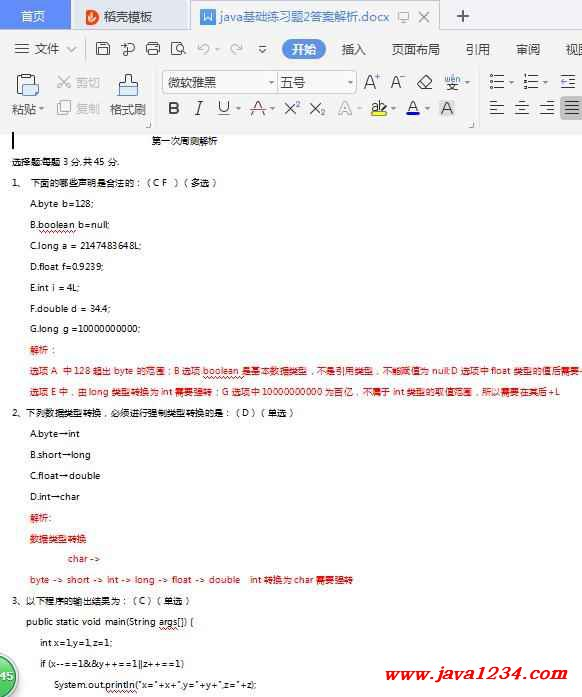Java知识分享网 - 轻松学习从此开始！

#Java1234官方群25：Java1234官方群25： 838462530

## java基础练习题2答案解析 PDF 下载

java基础练习题2答案解析 PDF 下载
 失效链接处理 java基础练习题2答案解析 PDF 下载 本站整理下载：   相关截图：主要内容： 选择题:每题3分,共45分. 1、 下面的哪些声明是合法的：（C F ）（多选）     A.byte b=128;      B.boolean b=null;      C.long a = 2147483648L;      D.float f=0.9239;      E.int i = 4L;      F.double d = 34.4; G.long g =10000000000; 解析： 选项A 中128超出byte的范围；B选项boolean是基本数据类型，不是引用类型，不能赋值为null;D选项中float类型的值后需要+f/F; 选项E中，由long类型转换为int需要强转；G选项中10000000000为百亿，不属于int类型的取值范围，所以需要在其后+L 2、下列数据类型转换，必须进行强制类型转换的是：（D）（单选）     A.byte→int      B.short→long      C.float→double      D.int→char      解析:     数据类型转换              char ->     byte -> short -> int -> long -> float -> double  int转换为char需要强转 3、以下程序的输出结果为：（C）（单选）    public static void main(String args[]) {       int x=1,y=1,z=1;       if (x--==1&&y++==1||z++==1)          System.out.println("x="+x+",y="+y+",z="+z);     }     A.x=0,y=1,z=1     B.x=0,y=2,z=2     C.x=0,y=2,z=1     D.x=1,y=2,z=1     详解：对于A&&B,如果a为假，就不判断B  为真，继续判断B       对于A||B,如果a为真，就不判断B  a为假，继续判断B       x--==1 为true，后为&&，则继续 y++==1 为true，后是||，因为之前为true，所以后续不再判断，不再执行z++==1了 4、以下代码的运行结果为：（C）（单选）   public class Calc {    public static void main (String args []) {       int total = 0;       for (int i = 0, j = 10; total > 30; ++i, --j) {           System.out.println(" i = " + i + " : j = " + j);           total += (i + j);       }       System.out.println("Total " + total);     }   }   A.产生运行错误   B.产生编译错误   C.输出 "Total 0"   D.产生如下输出 : i = 0 : j = 10 i = 1 : j = 9 i = 2 : j = 8 Total 30    解析：total值为0，不满足>30;所以不进入循环，直接执行后续代码 5、执行完以下代码int[] x=new int；后，下列各项正确的是：（A）（单选）  A.x为0 B.x未定义 C.x为0 D.x为空  详解：数组下标从0~length-1        总共25个元素  0-24        默认应该是0 6、下列Java标识符，错误的是：（DE）（多选）    A. _sys_varl     B. \$change     C. User_name       D. 1_file    E. car.taxi 详解：1.Java标示符的命名规定 1.以\$、_、字母开头，从第二个字符开始可以有数字。 2.大小写敏感。 3.不能与Java的关键字冲突。 7.在Java语言中，字符串“Java程序员”在内存中所占用的字节数是：（D）   A.10   B.7   C.13   D.14   解析：unicode字符集中，每个字符（包括中文，英文）占用2个字节，题中的字符串中共7个字符，所以共占用14个字节 8.下列代码段的输出结果是：（C）  public static void main(String[] args) {   int x = 12;   while (x < 10) {      x--;   }   System.out.print(x);  }   A.0   B.11   C.12   D.2  解析：考察while循环的执行顺序，先判断是否满足条件，不满足，不进入循环体 9.下列代码段中，循环执行的次数是：（C）   int x = 10;   do {      x--;   } while (x <=5);   A.10次    B.0次    C.1次    D.超过10次   详解：当初始情况不满足循环条件，while循环一次都不执行，          do-while循环不管任何情况都至少执行一次 10.下列代码输出结果是：（D） public class Foo {        public static void main(String[] args) {     int x = 5;     boolean b1 = true;     boolean b2 = false;     if((x==4)&&!b2)     System.out.println("1");     System.out.println("2");     if((b2=true)&&b1)     System.out.println("3");        } } A.2 B.3 C.1 2 3 D.2 3
------分隔线----------------------------